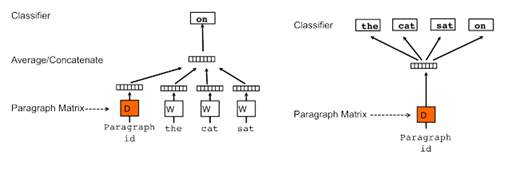• 3分钟热情学NLP第8篇，doc2vec计算句子相似度 word2vec面临的问题 word2vec计算句子或长文本的方法，大致的是： 1、对文本进行分词； 2、计算各个分词的词向量； 3、对词向量取平均值，或者其他方式进行词向量的...
3分钟热情学NLP第8篇，doc2vec计算句子相似度
word2vec面临的问题
word2vec计算句子或长文本的方法，大致的是： 1、对文本进行分词； 2、计算各个分词的词向量； 3、对词向量取平均值，或者其他方式进行词向量的拼接。
显而易见，这样的的计算方法的缺点是：丢失了文本之间的语序 比如：我喜欢小明，小明喜欢我。这2句话，语义不一样；但是通过上面的分词+词向量的方法，向量值是相同的。
因此，在word2vec的基础上，有研究人员提出了文本向量的概念doc2vec
2、doc2vec文本向量
Doc2vec方法是一种无监督算法，能从变长的文本（例如：句子、段落或文档）中学习得到固定长度的特征表示。Doc2vec也可以叫做 Paragraph Vector、Sentence Embeddings，它可以获得句子、段落和文档的向量表达，是Word2Vec的拓展，其具有一些优点，比如不用固定句子长度，接受不同长度的句子做训练样本。Doc2vec算法用于预测一个向量来表示不同的文档 ，该模型的结构潜在的克服了词袋模型的缺点。

参考：https://zhuanlan.zhihu.com/p/136096645

如下图所示：doc2vec算法
论文原文：http://cs.stanford.edu/~quocle/paragraph_vector.pdf
展开全文自然语言处理 nlp
• 为了能在聚类中更 准确地对文本进行相似度计算，本文采用 word2vec 将词语表示成词向量，并提出了一种基 10 于稠密特征的 DC-word2vec 算法，通过引入高频网络词组成的高维词表对特征向量进行扩维 映射，使其变得...
• X_train 就是自己的训练语料...doc2vec计算句子相似性 ”“” # coding:utf-8 import sys import time import csv import glob import gensim import sklearn import numpy as np import jieba.posseg as pseg imp...
X_train  就是自己的训练语料
“”“
date:2018_7_25
doc2vec计算句子相似性
”“”
# coding:utf-8

import sys
import time
import csv
import glob
import gensim
import sklearn
import numpy as np
import jieba.posseg as pseg
import jieba

from gensim.models.doc2vec import Doc2Vec, LabeledSentence

TaggededDocument = gensim.models.doc2vec.TaggedDocument

csvfile = open(path2,encoding='UTF-8')
stopwords  = [line.strip() for line in csvfile.readlines()]
return stopwords

def cut(data):
result=[]    #pos=['n','v']
res = pseg.cut(data)
list = []
for item in res:
#if item.word not in stop_words and (item.flag == 'n' or item.flag == 'a' or item.flag == 'v'):
if item.word not in stop_words :
list.append(item.word)
result.append(list)
return result

def get_all_content():
#abel_dir = [path + x for x in os.listdir(path) if os.path.isdir(path + x)]
all_files = glob.glob(r'D:/GFZQ/GFZQ/xuesu2018/xuesu/*.csv')
return all_files

def get_wenben(path):
csvfile = open(path,'r',encoding='UTF-8')

def get_QA(wenben):
Q_all =[]
A_all =[]
for QA in wenben :
Q_all.append(QA)
A_all.append(QA)
all = Q_all + A_all
return all,Q_all,A_all

def get_datasest(all_csv):
docs =  all_csv
print( len(docs))
x_train = []
# y = np.concatenate(np.ones(len(docs)))
all_sent = []
for file_one in docs:
for sent in file_one:
#print (sent)
all_sent.append(sent)
for i,text in enumerate(all_sent):
word_list = cut(text)
#print(word_list)
l = len(word_list)
print (l)
document = TaggededDocument(word_list, tags=[i])
x_train.append(document)
return x_train

def getVecs(model, corpus, size):
vecs = [np.array(model.docvecs[z.tags].reshape(1, size)) for z in corpus]
return np.concatenate(vecs)

def train(x_train, size=200, epoch_num=1):
model_dm = Doc2Vec(x_train, min_count=1, window=3, size=size, sample=1e-3, negative=5, workers=4)
model_dm.train(x_train, total_examples=model_dm.corpus_count, epochs=70)
model_dm.save('G:/project/sentimation_analysis/data/conference.model')
return model_dm

def get_csvfile ():
all_files = get_all_content()
length = 28  # len(all_files)
print ("统计了%d家公司的情感词" %length)
all_csv = []
for i in range(length):
print ("正在解析第%d家公司" %i)
file_one = all_files[i]
wenben = get_wenben(file_one)
all, Q_all, A_all = get_QA(wenben)
all_csv.append(all)
return all_csv

def stest():
test_text = ["我们是好孩子"]
inferred_vector_dm = model_dm.infer_vector(test_text)
# print  (inferred_vector_dm)
sims = model_dm.docvecs.most_similar([inferred_vector_dm], topn=10)
return sims

if __name__ == '__main__':

start = time.clock()
all_csv = get_csvfile()
x_train = get_datasest(all_csv)
model_dm = train(x_train)
sims = stest()
for count, sim in sims:
sentence = x_train[count]
print  ( sentence, sim, )


展开全文• 3分钟热情学NLP第7篇，Wrod2vec计算句子相似度实战 方法1，计算句子中各个词向量，得出句子的平均值 1、使用jieba分词，得出该句子包含的词； 2、计算每个词的词向量； 3、求得该句子的平均值 4、采用余弦值，计算...
3分钟热情学NLP第7篇，Wrod2vec计算句子相似度实战
方法1，计算句子中各个词向量，得出句子的平均值
1、使用jieba分词，得出该句子包含的词； 2、计算每个词的词向量； 3、求得该句子的平均值 4、采用余弦值，计算各个句子的相似度；
输入：
import numpy as np
from scipy import spatial
import jieba

model_dimension = 256 #该模型的维度为256维

#采用jieba进行分词，分词后计算每个词的词向量，最后求平均值；
def avg_string_vector(sentence, model):
words = jieba.cut(sentence)#默认jieba精确分词模式
string_vec = np.zeros((model_dimension,), dtype='float32')#返回1个"1*256"，元素为0的数组；
num_words = 0 #该句话中词语的个数，初始值为0
for word in words:
if word in model.wv:
num_words += 1#计算出该句话中，词语的个数

if(num_words > 0):
string_vec = np.divide(string_vec, num_words)#求平均值：将词向量相加后的和，除以分词的个数；
return string_vec

s1 = '在微信公众号上写文章'
s2= '把文章发表在微信公众号上'
s3 = '在微信上发表文章'
s4 = '欢迎大家来到微信总部参观'
s1_vec = avg_string_vector(s1, model=model)
s2_vec = avg_string_vector(s2, model=model)
s3_vec = avg_string_vector(s3, model=model)
s4_vec = avg_string_vector(s4, model=model)

#计算余弦距离
sim12 = 1 - spatial.distance.cosine(s1_vec, s2_vec)
sim13 = 1 - spatial.distance.cosine(s1_vec, s3_vec)
sim14 = 1 - spatial.distance.cosine(s1_vec, s4_vec)

print('”'+ s1 +'“和”' + s2 +'“的相似度为：%.4f'%sim12)
print('”'+ s1 +'“和”' + s3 +'“的相似度为：%.4f'%sim13)
print('”'+ s1 +'“和”' + s4 +'“的相似度为：%.4f'%sim14)

输出
”在微信公众号上写文章“和”把文章发表在微信公众号上“的相似度为：0.9013
”在微信公众号上写文章“和”在微信上发表文章“的相似度为：0.8178
”在微信公众号上写文章“和”欢迎大家来到微信总部参观“的相似度为：0.6783


方法2，使用第6篇提到的WMD算法
第6篇：https://www.jianshu.com/p/39e0c9d35f6c
代码如下：
import gensim
import pyemd

s1 = '在微信公众号上写文章'
s2= '把文章发表在微信公众号上'
s3 = '在微信上发表文章'
s4 = '欢迎大家来到微信总部参观'

#使用WMD距离算法
distance12 = model.wv.wmdistance(s1, s2)
distance13 = model.wv.wmdistance(s1, s3)
distance14 = model.wv.wmdistance(s1, s4)

print('”'+ s1 +'“和”' + s2 +'“的相似度为：%.4f'%distance12)
print('”'+ s1 +'“和”' + s3 +'“的相似度为：%.4f'%distance13)
print('”'+ s1 +'“和”' + s4 +'“的相似度为：%.4f'%distance14)

输出：
”在微信公众号上写文章“和”把文章发表在微信公众号上“的相似度为：0.7265
”在微信公众号上写文章“和”在微信上发表文章“的相似度为：1.1910
”在微信公众号上写文章“和”欢迎大家来到微信总部参观“的相似度为：2.2488


展开全文nlp 自然语言处理 算法
• 使用 gensim 训练中文词向量，计算词语之间的相似度。 输入：语料库，txt文件。 输出：余弦相似度。 实现代码： ...# -*- coding: utf-8 -*- ...from gensim.models import word2vec def main(): logging.basicCo...
使用 gensim 训练中文词向量，计算词语之间的相似度。
输入：语料库，txt文件。
输出：余弦相似度。
实现代码：
# -*- coding: utf-8 -*-

import logging

from gensim import models
from gensim.models import word2vec

def main():
logging.basicConfig(format='%(asctime)s : %(levelname)s : %(message)s', level=logging.INFO)
sentences = word2vec.LineSentence("output.txt")
model = word2vec.Word2Vec(sentences, size=250)

# 保存模型，供以后使用
model.save("word2vec.model")

# 模型读取

logging.basicConfig(format='%(asctime)s : %(levelname)s : %(message)s', level=logging.INFO)

print("提供 3 种测试模式\n")
print("输入一个词，则去寻找前一百个该词的相似词")
print("输入两个词，则去计算两个词的余弦相似度")
print("输入三个词，进行类比推理")

while True:
try:
query = input('')
q_list = query.split()

if len(q_list) == 1:
print("相似词前 100 排序")
res = model.most_similar(q_list, topn=100)
for item in res:
print(item + "," + str(item))

elif len(q_list) == 2:
print("计算 Cosine 相似度")
res = model.similarity(q_list, q_list)
print(res)
else:
print("%s之于%s，如%s之于" % (q_list, q_list, q_list))
res = model.most_similar([q_list, q_list], [q_list], topn=100)
for item in res:
print(item + "," + str(item))
print("----------------------------")
except Exception as e:
print(repr(e))

if __name__ == "__main__":
main()

gensim的参数解释：
from gensim.models import Word2Vec
model = Word2Vec(sentences, sg=1, size=100, window=5, min_count=5, negative=3, sample=0.001, hs=1, workers=4)
sg=1是skip-gram算法，对低频词敏感；默认sg=0为CBOW算法。size是输出词向量的维数，值太小会导致词映射因为冲突而影响结果，值太大则会耗内存并使算法计算变慢，一般值取为100到200之间。window是句子中当前词与目标词之间的最大距离，3表示在目标词前看3-b个词，后面看b个词（b在0-3之间随机）。min_count是对词进行过滤，频率小于min-count的单词则会被忽视，默认值为5。negative和sample可根据训练结果进行微调，sample表示更高频率的词被随机下采样到所设置的阈值，默认值为1e-3。hs=1表示层级softmax将会被使用，默认hs=0且negative不为0，则负采样将会被选择使用。workers控制训练的并行，此参数只有在安装了Cpython后才有效，否则只能使用单核。 
展开全文Python
• 在生成词向量之后，基于词向量计算句子相似度： 1.首先处理句子的重点词汇，排除无关词汇，比如我你他之类的，non_words.txt里面是一些无关词汇 2.接下来根据相关的处理结果，做一个单项匹配 首先根据s1的句子...
• 3分钟热情学NLP第6篇，Wrod2vec计算句子相似度 参考文章： 1、https://blog.csdn.net/joleoy/article/details/99741139 2、https://www.zhihu.com/question/29978268 1、无监督，句子相似度的计算方法 无监督，即...算法 自然语言处理 nlp
• 词语相似度计算 在商品搜索的过程中，可以计算用户输入的关键字与数据库中商品名间的相似度，在商品...在实际的工程开发中可以通过word2vec实现词语相似度计算。 from sklearn.datasets import fetch_20newsgr...
• 2.7 Word2Vec与文章相似度 学习目标 目标 知道文章向量计算方式 了解Word2Vec模型原理 知道文章相似度计算方式 应用 应用Spark完成文章相似度计算 2.7.1 文章相似度 在我们的头条推荐中有很多地方...人工智能 推荐系统
• 关注公众号，一起学算法作者：刘才权编辑：黄俊嘉基于word2vec的词语相似度计算应用场景假设你有一个商品的数据库，比如：现在通过用户的输入来检索商品的价格，最简单的方法就是通过字符串进行匹配，比如，用户输入...
• Google开源word2vec，文本相似度计算工具 谷歌已经使用Deep Learning技术开发了许多新方法来解析语言，目前，谷歌开源了一款基于Deep Learning的学习工具——word2vec，这是首款面向大众的Deep Learning学习工具...
• 运用Python+Word2vec实现文本相似度 // An highlighted block import pymssql import pandas as pd import jieba #from gensim import corpora, models,similarities #import numpy as np #import nltk from...Python 文本挖掘 推荐算法
• 深度学习，人工智能，自然语言处理，机器学习，深度学习自然语言处理
• @基于doc2vec计算文本相似度 Doc2vec ​Doc2vec又叫Paragraph Vector是Tomas Mikolov基于word2vec模型提出的，其具有一些优点，比如不用固定句子长度，接受不同长度的句子做训练样本，Doc2vec是一个无监督学习算法，...机器学习 算法 自然语言处理
• 采用维基百科里的中文网页作为训练语料库，下载地址为：https://dumps.wikipedia.org/zhwiki/20190301/zhwiki-20190301-pages-articles.xml.bz2 维基百科提供的语料是xml格式的，因此需要将其转换为txt格式。由于...
• 在python中，如何使用word2vec计算句子相似度呢？ 第一种解决方法 如果使用word2vec，需要计算每个句子/文档中所有单词的平均向量，并使用向量之间的余弦相似度计算句子相似度，代码示例如下 import numpy as ...
• Doc2Vec模型基于Word2vec模型，并在其基础上增加了一个段落向量。 以Doc2Vec的C-BOW方法为例。算法的主要思想在以下两个方面： 训练过程中新增了paragraph id，即训练语料中每个句子都有一个唯一的id。paragraph id...向量空间模型 gensim NLP
• ## doc2vec计算文档相似度

万次阅读 多人点赞 2016-09-07 16:47:14
doc2vec是基于word2vec的，word2vec对于计算两个词语的相似度效率比较好，修改了word2vec中的cbow和skip-gram模型，paragraph vector直接得到doc向量。 使用过程 1.读取文件，os.walk()遍历文件夹 2.构建语料库，...
• 在python中，如何使用word2vec计算句子相似度呢？ 第一种解决方法 如果使用word2vec，需要计算每个句子/文档中所有单词的平均向量，并使用向量之间的余弦相似度计算句子相似度，代码示例如下： import ...
• 最近在做判断两个文本是否是描述的同一件事情，之前是采用gensim中的TF-IDF进行计算的...我们都知道word2vec是可以分析语义的，那么doc2vec是不是也可以分析出语义呢？于是试了一下gensim中的doc2vec。 Doc2Vec 也...gensim python
• #提取关键词#关键词向量化#相似度计算from jieba import analyseimport numpyimport gensim# 实现给出任意字符串，获取字符串中某字符的位置以及出现的总次数def get_char_pos(string,char): chPos=[] try: chPos=...
• ## 用gensim doc2vec计算文本相似度

万次阅读 热门讨论 2017-07-14 16:48:14
最近开始接触gensim库，之前训练word2vec用Mikolov的c版本程序，看了很久才把程序看明白，在gensim库中，word2vec和doc2vec只需要几个接口就可以实现，实在是方便。python，我越来越爱你了。 这个程序很简单，直接...
• 使用python语言使用word2vec 的方法来进行语义相似度计算python
• __author__ = 'su' import os import logging import sys import re import jieba import multiprocessing import gensim ...from gensim.corpora import WikiCorpus...from gensim.models import Word2Vec from gens...
• 一.中文语料库 中文语料库主要有以下几种： 1. 腾讯AI Lab2018年分享的开源800万中文词的NLP数据集文章...mid=2247506252&idx=4&sn=1f883532975737c9df02212fc2ae1ca5&chksm=e8d06e3edf...
• ## 计算文本相似度－Word2Vec计算

千次阅读 热门讨论 2018-10-18 19:14:47
Word2Vec，顾名思义，其实就是将每一个词转换为向量的过程。 如果不了解的话可以参考：https://blog.csdn.net/itplus/article/details/37969519 这里我们可以直接下载训练好的 Word2Vec 模型， 模型的链接地址为...
• """Basic word2vec example.""" from __future__ import absolute_import from __future__ import division from __future__ import print_function import matplotlib matplotlib.use('Agg') #jason import ......